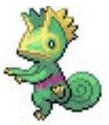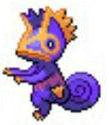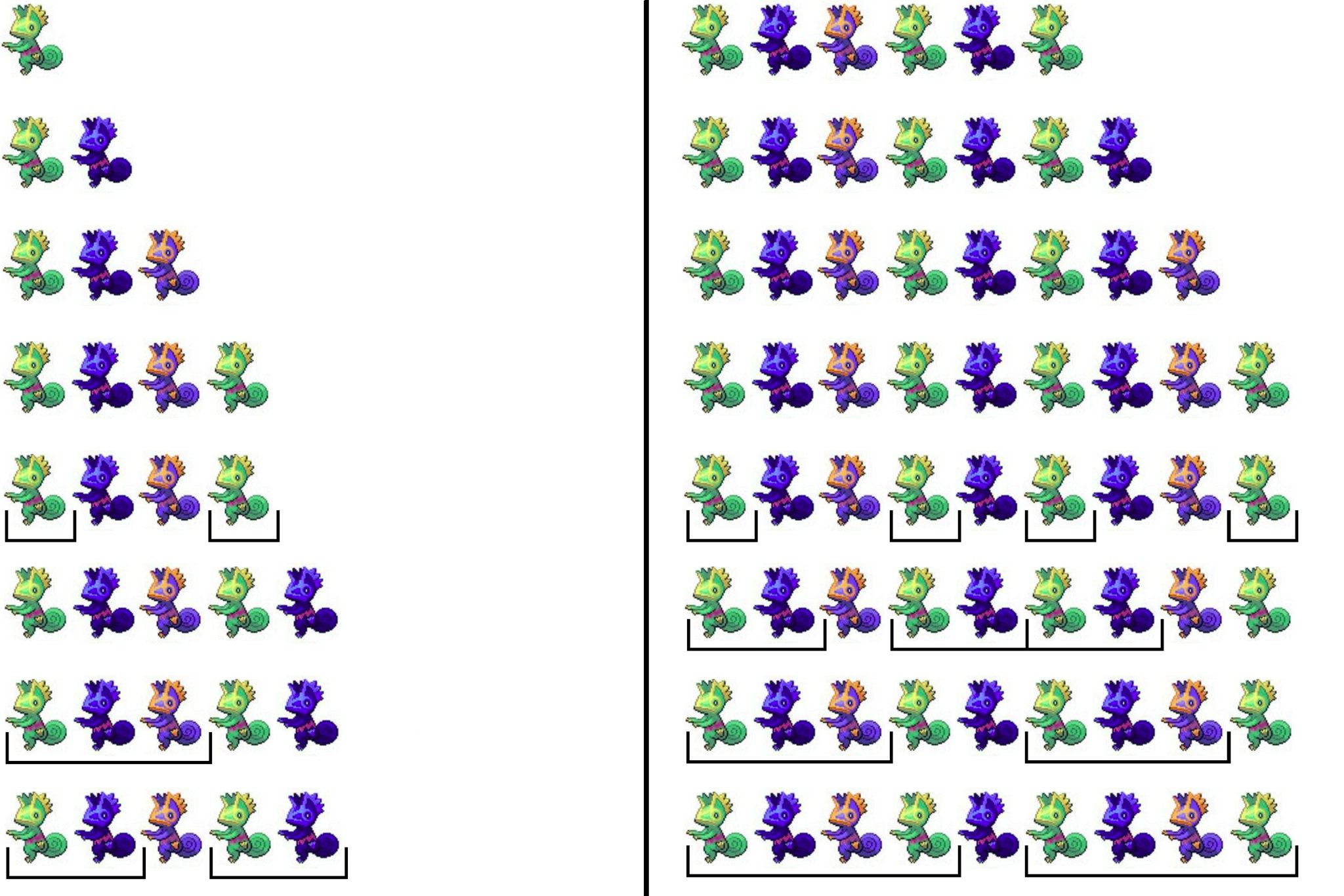시간 제한 메모리 제한 제출 정답 맞은 사람 정답 비율
4 초 512 MB 4 3 3 100.000%

## 문제

Do you know how Kecleon looks? Similar to chameleons, its skin can be colored in various ways and this is what this task is about. For the purposes of this problem, let’s assume that the body of Kecleon is colored in one out of 26 different colors, each denoted by a distinct lowercase English character.

Multiple Kecleon want to form a line, which is initially empty. You have to process two types of queries:

• In the first type, a Kecleon of a specified color comes to the right end of the line and remains there forever.
• In the second type, you’re given an integer k. For each contiguous interval of k specimens in the line, consider the sequence of colors of Kecleon in this interval, from left to right. You have to say how many of these sequences are exactly the same as the sequence of colors of the first k Kecleon in the line.

Unfortunately, Kecleon still don’t know the order in which they will join the line. Therefore, you need to process the queries online.

## 입력

The input is encoded. In order to decode the queries, you need to maintain a variable last which is initially equal to 0. After each query of the second type, the value of last is set to the most recently computed answer.

The first line contains one integer q (2 ≤ q ≤ 300 000) — the number of queries in the input.

Each of the next q lines contains a description of one query. Each is in one of the following formats:

• add ci′ (ci′ is a lowercase English letter) — Let ci = char((asc(ci′) + last) mod 26) where asc(x) denotes the 0-based index of x in the English alphabet, and char(x) means the x-th character in the English alphabet (0-based as well). This operation adds a Kecleon of color ci to the right end of the line.
• get ki′ (1 ≤ ki′ ≤ n) — Let n be equal to the number of Kecleon currently in the line. Now, let ki = ((ki′ − 1 + last) mod n) + 1. You need to find out how many intervals of length ki have the same sequence of colors as the first ki Kecleon in the line.

It’s guaranteed that the first query has type add and that there is at least one query of type get.

## 출력

For each query of type get output one line containing one integer described in the statement.

## 예제 입력 1

16
get 1
get 1
get 1
get 8
get 7
get 9
get 2


## 예제 출력 1

2
1
2
4
3
2
2


## 힌트

In this sample, Kecleon come to the line in the following order: abcababca. The decoded values ki are: 1, 3, 2, 1, 2, 3, and 4.

Assume that ‘a’ =, ‘b’ =and ‘c’ =. Here is the exact order of queries: